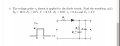# output of half-wave rectifier with voltage sources

#### MHillis

Joined Oct 25, 2014
6
I could use some help on how best to approach a problem like this. The diode is ideal. My attempt so far is:

1. Before the pulse the diode is off because of the 10 volt source. Then its just a series resistor and capacitor. At steady state all ten volts are across the capacitor and current is zero.

2.So after the pulse the same thing should happen with v2 going to 10 volts.

3. During the pulse there's a new three volts on one side of the diode. But I don't see how that is enough to turn the diode on. If the diode doesn't turn on the circuit can't change.

I'm not really sure where to go from here. Any help would be greatly appreciated.#### shteii01

Joined Feb 19, 2010
4,644
At what voltage does diode turn On?

#### MHillis

Joined Oct 25, 2014
6
It should turn on when there is a higher potential on the V1 side than on the V2 side

#### MikeML

Joined Oct 2, 2009
5,444
Nothing happens is a possible answer. Is it the right one?

#### MHillis

Joined Oct 25, 2014
6
I wish I knew. We don't have any solutions manuals for our homework questions.

#### t_n_k

Joined Mar 6, 2009
5,455
Just a thought - Perhaps one has to assume the capacitor is uncharged at t=0. Then there may be something more interesing to analyze.

#### MrChips

Joined Oct 2, 2009
21,090
You have to make the assumption that the capacitor is uncharged at t = 0.
Also the diode is an ideal diode that conducts when forward biased.

Do you know the formula for an RC charging circuit?

#### MHillis

Joined Oct 25, 2014
6
You have to make the assumption that the capacitor is uncharged at t = 0.
Also the diode is an ideal diode that conducts when forward biased.

Do you know the formula for an RC charging circuit?
So correct me if I'm approaching this the wrong way. The capacitor is uncharged at t=0. There's basically two circuits, one before the capacitor charges to 3 volts and one after. Before the capacitor charges to 3 volts, both the 3 volt source and 10 volt source charge the capacitor at some time constant rate. After it is just the 10 volt source charging the capacitor.

#### t_n_k

Joined Mar 6, 2009
5,455
So correct me if I'm approaching this the wrong way. The capacitor is uncharged at t=0. There's basically two circuits, one before the capacitor charges to 3 volts and one after. Before the capacitor charges to 3 volts, both the 3 volt source and 10 volt source charge the capacitor at some time constant rate. After it is just the 10 volt source charging the capacitor.
That's correct.

#### MHillis

Joined Oct 25, 2014
6
Ok, so from using a laplace transform on the circuit I'm seeing that were there no diode, the capacitor would charge up to the average of the two voltages. Is that the correct behavior? The second part of the circuit charges to 10 volts as expected.

#### t_n_k

Joined Mar 6, 2009
5,455
Ok, so from using a laplace transform on the circuit I'm seeing that were there no diode, the capacitor would charge up to the average of the two voltages. Is that the correct behavior? The second part of the circuit charges to 10 volts as expected.
Yes - again correct. Mind you, you should be able to readily deduce the final value with the diode removed without needing Laplace transform.

#### MrAl

Joined Jun 17, 2014
7,590
Ok, so from using a laplace transform on the circuit I'm seeing that were there no diode, the capacitor would charge up to the average of the two voltages. Is that the correct behavior? The second part of the circuit charges to 10 volts as expected.

Hello there,

It is true that for this circuit you might consider the voltage charging to the average voltage of the two when the diode is shorted, but i dont think you should look at it that way because if one of the resistors was not 10 ohms (like one 10 and one 25 ohms) then you'd want to know how to handle that case too.

One way is to short the diode and then handle it as a two source circuit, perhaps using superposition. You could then solve for the *time* when the cap charges up to 3 volts because that is the time when the diode would stop conducting if the pulse was infinitely long. But if that time comes out longer than the pulse, then you only need to allow that response up to that time period.
Once you have that solution (time and voltage) you can then move on to the next phase.
Next you use the voltage you found as the initial voltage of the capacitor and the new circuit with just the 10v source to find the remainder of the solution.
The total solution will be the first phase for that time period and then the second phase follows that for the remainder of the time.
The entire output should look like exponentials defined over two different periods of time.

You can use Laplace if you like unless you know the formulas for a charging capacitor through a resistor with and without some initial cap voltage, and dont forget the second phase has an initial cap voltage as it does not start out at zero then.

#### WBahn

Joined Mar 31, 2012
25,751
Why is everyone wanting to assume that the capacitor is uncharged at time t=0? It isn't!

The circuit is well defined for all time t > -00. At t=0- it is in steady state with V1=0V. What does V2 have to be?